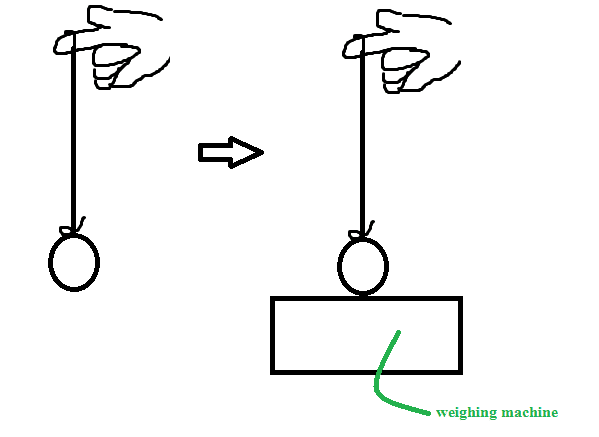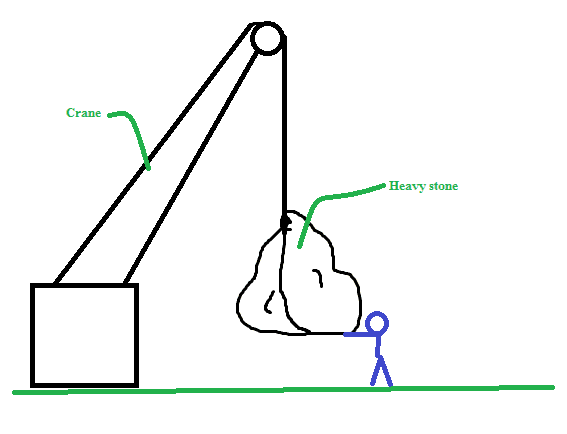# Mechanics Pulley Platform Problem

## Homework Statement

Two construction workers each of mass m raise themselves on a hanging platform using pulleys as shown above. If the platform has a mass of 1.2⋅m, the initial distance between the pulleys and the platform is d, and the workers each pull with a force f on the ropes, what is the acceleration a of the workers? Assume the pulleys and ropes are massless.[/B]

## Homework Equations

Tension = m(a + g) (possibly).
F = ma[/B]

## The Attempt at a Solution

To be wholly honest I have next to no idea how to proceed. My only real thought was to use F = ma. Thinking first that I'd need my total mass, I added (m + m + 1.2m) = 3.2m total (one mass for each worker plus the platform). Next I tried to consider the force, thinking we have two workers each exerting a tension force f, thus 2f being the total force upwards, then subtracting out the downward forces (namely gravity) to achieve (2f - 3.2mg). Plugging into F = ma I got (2f - 3.2mg) = a(3.2m) => a = (2f - 3.2mg)/(3.2m) which is marked incorrect. At this point I have hit a wall. My thanks for any help.

(Attached is the image accompanying the problem).
[/B]

#### Attachments

•Men_and_pulleys.png
1.6 KB · Views: 476
Last edited:
•arpon

arpon
(2f - 3.4mg)
Why 3.4mg ?

Sorry, that was a typo which persisted through my idiocy. I corrected it.

•arpon
arpon
I suggest you to think about the free body diagram.
At first, think about the free body diagram of one of the men.
He is exerting force f each on the rope, and so according to Newton's third law, the rope is also exerting force f (upward) on him. Let's assume, he is exerting a force R on the platform, and the platform is exerting the same force R on him in the upward direction. The other force on him is gravitational force which is equal to mg. So the net force on him is, ##F_{net} = R + f - mg ##; Again, ##F_{net} = ma##. So ## R + f - mg = ma##
In this same way, you may find out, equations for the other man and the platform, and solving these equations, you may find out accelaration.

Wouldn't the force R simply be equal the normal force the man is exerting on the platform and in turn then be equal to ##mg##, giving ##f = ma## out again since R would cancel with ##mg##.

arpon
Wouldn't the force R simply be equal the normal force the man is exerting on the platform and in turn then be equal to ##mg##, giving ##f = ma## out again since R would cancel with ##mg##.
No. Suppose, you hang a ball with a rope. The rope is straight. Then you just touch the ball on a weighing machine keeping the rope straight. Will there be any weight found on the machine?arpon
Another example. Suppose, a heavy stone hangs from a crane. And you touch your hand under the stone. Will you feel any force on your hand?Alright, I think I understand what you mean.

So would the equation for the other man just be ##R+f−mg=ma## since he is identical to the first man and the equation for platform would be ##2f -1.2mg = ma## Two f for the tensions forces acting on the block, minus the downward component of force?

I'll work on it more tomorrow as well, if I can't reply any more tonight.

arpon
the equation for platform would be 2f−1.2mg=ma2f -1.2mg = ma
You are missing the normal forces which are exerted on the platform by the men, and look, the mass of the platform is not m. When you are calculating with the forces on the platform, the net force equals to the mass of 'the platform' times the accelaration of 'the platform'.

So it would then be: ##2f - 1.2mg - 2R = 1.2mg## (because since before you defined the force R to be the force the platform exerts on the men upwards, the normal force for the platform by the men should be twice the negative of R).

arpon
: 2f−1.2mg−2R=1.2mg
"## = 1.2mg##" ? Probably, a typo.

Should have been ##=1.2ma## I think.

•arpon
•